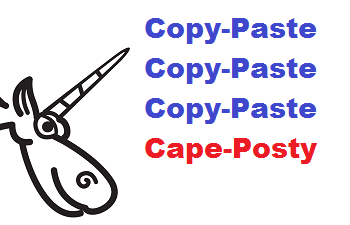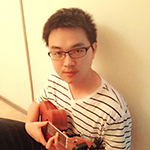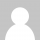# 编程中的“末行效应”## 末行效应

```inline Vector3int32& operator+=(const Vector3int32& other) {
x += other.x;
y += other.y;
z += other.y;
return *this;
}

```

```strncmp(argv[argidx], "CAT=", 4) &&
strncmp(argv[argidx], "DECOY=", 6) &&
strncmp(argv[argidx], "MINPROB=", 8)) {
```

## 实例：

### Source Engine SDK

```inline void Init( float ix=0, float iy=0,
float iz=0, float iw = 0 )
{
SetX( ix );
SetY( iy );
SetZ( iz );
SetZ( iw );
}
```

### Chromium

```if (access & FILE_WRITE_ATTRIBUTES)
output.append(ASCIIToUTF16("\tFILE_WRITE_ATTRIBUTES\n"));
if (access & FILE_WRITE_DATA)
output.append(ASCIIToUTF16("\tFILE_WRITE_DATA\n"));
if (access & FILE_WRITE_EA)
output.append(ASCIIToUTF16("\tFILE_WRITE_EA\n"));
if (access & FILE_WRITE_EA)
output.append(ASCIIToUTF16("\tFILE_WRITE_EA\n"));
break;
```

### ReactOS

```if (*ScanString == L'\"' ||
*ScanString == L'^' ||
*ScanString == L'\"')

```

### Multi Theft Auto

```class CWaterPolySAInterface
{
public:
WORD m_wVertexIDs;
};
{
....
pInterface->m_wVertexIDs [ 0 ] = pV1->GetID ();
pInterface->m_wVertexIDs [ 1 ] = pV2->GetID ();
pInterface->m_wVertexIDs [ 2 ] = pV3->GetID ();
pInterface->m_wVertexIDs [ 3 ] = pV4->GetID ();
....
}
```

### Source Engine SDK

```intens.x=OrSIMD(AndSIMD(BackgroundColor.x,no_hit_mask),
```

### Trans-Proteomic Pipeline

```void setPepMaxProb(....)
{
....
double max4 = 0.0;
double max5 = 0.0;
double max6 = 0.0;
double max7 = 0.0;
....
if ( pep3 ) { ... if ( use_joint_probs && prob > max3 ) ... }
....
if ( pep4 ) { ... if ( use_joint_probs && prob > max4 ) ... }
....
if ( pep5 ) { ... if ( use_joint_probs && prob > max5 ) ... }
....
if ( pep6 ) { ... if ( use_joint_probs && prob > max6 ) ... }
....
if ( pep7 ) { ... if ( use_joint_probs && prob > max6 ) ... }
....
}
```

### SeqAn

```inline typename Value<Pipe>::Type const & operator*() {
tmp.i1 = *in.in1;
tmp.i2 = *in.in2;
tmp.i3 = *in.in2;
return tmp;
}
```

### SlimDX

```for( int i = 0; i < 2; i++ )
{
sliders[i] = joystate.rglSlider[i];
asliders[i] = joystate.rglASlider[i];
vsliders[i] = joystate.rglVSlider[i];
fsliders[i] = joystate.rglVSlider[i];
}
```

### Qt

```if (repetition == QStringLiteral("repeat") ||
repetition.isEmpty()) {
pattern->patternRepeatX = true;
pattern->patternRepeatY = true;
} else if (repetition == QStringLiteral("repeat-x")) {
pattern->patternRepeatX = true;
} else if (repetition == QStringLiteral("repeat-y")) {
pattern->patternRepeatY = true;
} else if (repetition == QStringLiteral("no-repeat")) {
pattern->patternRepeatY = false;
pattern->patternRepeatY = false;
} else {
//TODO: exception: SYNTAX_ERR
}

```

```pattern->patternRepeatX = false;
pattern->patternRepeatY = false;
```

### ReactOS

```const int istride = sizeof(tmp) / sizeof(tmp);
const int jstride = sizeof(tmp) / sizeof(tmp);
const int mistride = sizeof(mag) / sizeof(mag);
const int mjstride = sizeof(mag) / sizeof(mag);
```

‘mjstride’永远等于1。最后一行应该是：

```const int mjstride = sizeof(mag) / sizeof(mag);
```

### Mozilla Firefox

```if (protocol.EqualsIgnoreCase("http") ||
protocol.EqualsIgnoreCase("https") ||
protocol.EqualsIgnoreCase("news") ||
protocol.EqualsIgnoreCase("ftp") ||          <<<---
protocol.EqualsIgnoreCase("file") ||
protocol.EqualsIgnoreCase("javascript") ||
protocol.EqualsIgnoreCase("ftp")) {          <<<---
```

### Quake-III-Arena

```if (fabs(dir) > test->radius ||
```

dir的值忘记检查了。

### Clang

```return (ContainerBegLine <= ContaineeBegLine &&
ContainerEndLine <= ContaineeEndLine &&
(ContainerBegLine != ContaineeBegLine ||
SM.getExpansionColumnNumber(ContainerRBeg) <=
SM.getExpansionColumnNumber(ContaineeRBeg)) &&
(ContainerEndLine != ContaineeEndLine ||
SM.getExpansionColumnNumber(ContainerREnd) >=
SM.getExpansionColumnNumber(ContainerREnd)));
```

### MongoDB

```bool operator==(const MemberCfg& r) const {
....
h == r.h && priority == r.priority &&
arbiterOnly == r.arbiterOnly &&
slaveDelay == r.slaveDelay &&
hidden == r.hidden &&
buildIndexes == buildIndexes;
}
```

### Unreal Engine 4

```static bool PositionIsInside(....)
{
return
Position.X >= Control.Center.X - BoxSize.X * 0.5f &&
Position.X <= Control.Center.X + BoxSize.X * 0.5f &&
Position.Y >= Control.Center.Y - BoxSize.Y * 0.5f &&
Position.Y >= Control.Center.Y - BoxSize.Y * 0.5f;
}
```

### Qt

```qreal x = ctx->callData->args.toNumber();
qreal y = ctx->callData->args.toNumber();
qreal w = ctx->callData->args.toNumber();
qreal h = ctx->callData->args.toNumber();
if (!qIsFinite(x) || !qIsFinite(y) ||
!qIsFinite(w) || !qIsFinite(w))

```

### OpenSSL

```if (!strncmp(vstart, "ASCII", 5))
arg->format = ASN1_GEN_FORMAT_ASCII;
else if (!strncmp(vstart, "UTF8", 4))
arg->format = ASN1_GEN_FORMAT_UTF8;
else if (!strncmp(vstart, "HEX", 3))
arg->format = ASN1_GEN_FORMAT_HEX;
else if (!strncmp(vstart, "BITLIST", 3))
arg->format = ASN1_GEN_FORMAT_BITLIST;

```

## 结论

[英文原文：The Last Line Effect ]

### 本文的译者：Ryan Chen

Ryan Chen(英文名)。目前在美国圣地亚哥，高通高级工程师。他的微博是@奋斗中的胖胖。你还可以通过邮箱ryanmailing@gmail.com和他进行交流。### 11 Responses to 编程中的“末行效应”

1. rigid  这篇文章
2. 周正 对这篇文章的反应是垃圾
3.周正 says:

我很仔细，没出现过这种低级错误

4.我的 says:

差不多吧.但是改正之后就会出现相反效果

5. 王然  这篇文章
6.王然 says:

确实如此，复制-粘贴太容易出错了，所以一定要小心！

7. luobtang 对这篇文章的反应是俺的神呀
8.黄先生 says:

一龙耗材(www.nnyl.cn)是广西南宁最大的办公用品、办公设备、办公耗材批发与维修公司，是政府单位定点采购供应商。提供一条龙服务，免费送货、免费维修、免费加粉。主营惠普、联想、三星等品牌的办公用纸，打印机，复印机，墨盒，硒鼓等几万种办公用品以及耗材。热线电话：0771-2286966

9.翼发云 says:

免费的在线敏捷项目管理工具，翼发云IT项目管理系统，详见：http://www.effapp.com/sepm/

10.qwe520 says:

地标金融P2P理财平台：http://www.dib66.com

11.xinwendashibaike says:

这个确实应该是要注意，越是最后越容易忽视